Request a Free Counselling Session from our Expert Mentor# NCERT Solution for Class 12 Mathematics Chapter 7

NCERT Solution for Class 12 Mathematics Chapter 7, “Integrals,” is an essential study material designed to help students understand the fundamental concepts of integral calculus. Swastik Classes, a leading coaching institute, has developed comprehensive NCERT solutions that provide step-by-step explanations and solved examples to help students develop a deeper understanding of the subject. The chapter covers topics such as properties of integrals, methods of integration, integration using partial fractions, and integration using trigonometric identities. With the help of Swastik Classes’ NCERT solutions, students can improve their problem-solving skills and gain the confidence to tackle complex integral problems. These solutions are also useful for students who are preparing for competitive exams like JEE, NEET, and other entrance exams. Overall, Swastik Classes’ NCERT Solution for Class 12 Mathematics Chapter 7 is an essential resource for students who want to excel in mathematics and build a strong foundation in integral calculus.

## Answers of Mathematics NCERT solutions for class 12 Chapter 7 Integrals

Chapter 7

Integrals

#### Exercise-7.1

Question 1:

sin 2x

The anti-derivative of sin 2x is a function of x whose derivative is sin 2x.

It is known that,

Therefore, the anti-derivative of is

Question 2:

Cos 3x

The anti-derivative of cos 3x is a function of x whose derivative is cos 3x.

It is known that,

Therefore, the anti-derivative of  is .

Question 3:

e2x

The anti-derivative of e2is the function of x whose derivative is e2x.

It is known that,

Therefore, the anti-derivative of is

Question 4:

The anti-derivative of

is the function of whose derivative is

It is known that,

Therefore, the anti-derivative of is

Question 5:

The anti-derivative of  is the function of x whose derivative is .

It is known that,

Therefore, the anti-derivative of  is .

Question 6:

Question 7:

Question 8:

Question 9:

Question 10:

Question 11:

Question 12:

Question 13:

On dividing, we obtain

Question 14:

Question 15:

Question 16:

Question 17:

Question 18:

Question 19:

Question 20:

Question 21:

The anti-derivative of equals

(A) (B)

(C)  (D)

Hence, the correct answer is C.

Question 22:

If such that f(2) = 0, then f(x) is

(A)   (B)

(C)   (D)

It is given that,

∴Anti derivative of

Also,

Hence, the correct answer is A.

Exercise-7.2

Question 1:

Let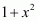t

∴2x dx = dt

Question 2:

Let log |x| = t

∴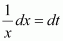Question 3:

Let 1 + log t

∴Question 4:

sin x ⋅ sin (cos x)

sin x ⋅ sin (cos x)

Let cos x = t

∴ −sin x dx = dt

Question 5:

Let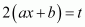Question 6:

Let ax + b = t

Question 7:

Let

∴ dx = dt

Question 8:

Let 1 + 2x2 = t

∴ 4xdx = dt

Question 9:

Let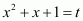∴ (2x + 1)dx = dt

Question 10:

Let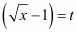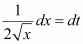Question 11:

Put

Question 12:

Let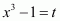∴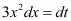Question 13:

Let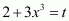∴ 9x2 dx = dt

Question 14:

Let log x = t

∴Question 15:

Let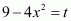∴ −8x dx = dt

Question 16:

Let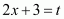∴ 2dx = dt

Question 17:

Let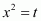∴ 2xdx = dt

Question 18:

Let

∴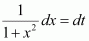Question 19:

Dividing numerator and denominator by ex, we obtain

Let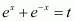∴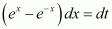Question 20:

Let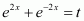∴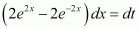Question 21:

Put

Question 22:

Let 7 − 4x = t

∴ −4dx = dt

Question 23:

Let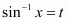∴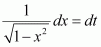Question 24:

Let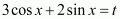∴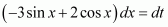Question 25:

Let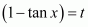∴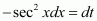Question 26:

Let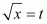∴Question 27:

Let sin 2x = t

∴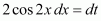Question 28:

Let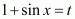∴ cos x dx = dt

Question 29:

cot x log sin x

Let log sin x = t

Question 30:

Let 1 + cos x = t

∴ −sin x dx = dt

Question 31:

Let 1 + cos x = t

∴ −sin x dx = dt

Question 32:

Let sin x + cos x = t

⇒ (cos x − sin xdx = dt

Question 33:

Put cos x − sin x = t

⇒ (−sin x − cos xdx = dt

*Question 34:

*Question 35:

Let 1 + log x = t

∴*Question 36:

Let

∴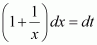*Question 37:

Let x4 = t

∴ 4x3 dx = dt

Let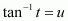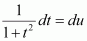From (1), we obtain

Question 38:

equals

Let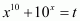∴Hence, the correct answer is D.

Question 39:

equals

A.  B.

C.  D.

Hence, the correct answer is B.

Exercise-7.3

Question 1:

Question 2:

It is known that,

Question 3:

cos 2x cos 4x cos 6x

It is known that,

Question 4:

sin3 (2x + 1)

Let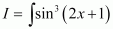Question 5:

sin3 x cos3 x

Question 6:

sin x sin 2x sin 3x

It is known that,

Question 7:

sin 4x sin 8x

It is known that,

sin A . sin B = 12cos(A-B)-cos(A+B)

∴∫sin4x sin8x dx

=∫12cos4x-8x-cos4x+8xdx

=12∫cos-4x-cos12xdx

=12∫cos4x-cos12xdx

=12sin4x4-sin12x12+C

Question 8:

Question 9:

Question 10:

sin4 x

Question 11:

cos4 2x

Question 12:

Question 13:

Question 14:

Question 15:

Question 16:

tan4x

From equation (1), we obtain

Question 17:

Question 18:

Question 19:

*Question 20:

*Question 21:

sin−1 (cos x)

It is known that,

Substituting in equation (1), we obtain

*Question 22:

*Question 23: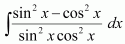is equal to

A.  tan x + cot x + C

B.  tan x + cosec x + C

C.  −tan x + cot x + C

D.  tan x + sec x + C

Hence, the correct answer is A.

*Question 24:

equals

A.

B.

C.

D.

Let (e^x).x = t

Hence, the correct answer is B.

Exercise-7.4

Question 1:

Let x3 = t

∴ 3x2 dx = dt

Question 2:

Let 2x = t

∴ 2dx = dt

Question 3:

Let 2 − t

⇒ −dx = dt

Question 4:

Let 5x = t

∴ 5dx = dt

Question 5:

Question 6:

Let x3 = t

∴ 3x2 dx = dt

Question 7:

From (1), we obtain

Question 8:

Let x3 = t

⇒ 3x2 dx = dt

Question 9:

Let tan x = t

∴ sec2x dx = dt

Question 10:

Question 11:

=

Let (3x+1)=t

∴3 dx = dt

=(1/3)

Question 12:

Question 13:

Question 14:

Question 15:

Question 16:

Equating the coefficients of x and constant term on both sides, we obtain

4A = 4 ⇒ A = 1

A + B = 1 ⇒ B = 0

Let 2x2 + x − 3 = t

∴ (4x + 1) dx dt

Question 17:

Equating the coefficients of x and constant term on both sides, we obtain

From (1), we obtain

From equation (2), we obtain

Question 18:

Equating the coefficient of x and constant term on both sides, we obtain

Substituting equations (2) and (3) in equation (1), we obtain

Question 19:

Equating the coefficients of x and constant term, we obtain

2A = 6 ⇒ A = 3

−9A + B = 7 ⇒ B = 34

∴ 6x + 7 = 3 (2x − 9) + 34

Substituting equations (2) and (3) in (1), we obtain

Question 20:

Equating the coefficients of x and constant term on both sides, we obtain

Using equations (2) and (3) in (1), we obtain

Question 21:

Let x2 + 2x +3 = t

⇒ (2x + 2) dx =dt

Using equations (2) and (3) in (1), we obtain

*Question 22:

Equating the coefficients of x and constant term on both sides, we obtain

Substituting (2) and (3) in (1), we obtain

*Question 23:

Equating the coefficients of x and constant term, we obtain

Using equations (2) and (3) in (1), we obtain

*Question 24:

equals

A.  x tan−1 (x + 1) + C

B.  tan− 1 (x + 1) + C

C.  (x + 1) tan−1 x + C

D.  tan−1 x + C

Hence, the correct answer is B.

*Question 25: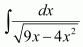equals

A.

B.

C.

D.

Hence, the correct answer is B.

Exercise-7.5

Question 1:

Let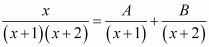Equating the coefficients of x and constant term, we obtain

A + = 1

2A + B = 0

On solving, we obtain

A = −1 and B = 2

Question 2:

Let

Equating the coefficients of x and constant term, we obtain

A + B = 0

−3A + 3B = 1

On solving, we obtain

Question 3:

LetSubstituting x = 1, 2, and 3 respectively in equation (1), we obtain

A = 1, B = −5, and C = 4

Question 4:

Let

Substituting x = 1, 2, and 3 respectively in equation (1), we obtain  and

Question 5:

Let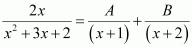Substituting x = −1 and −2 in equation (1), we obtain

A = −2 and B = 4

Question 6:

It can be seen that the given integrand is not a proper fraction.

Therefore, on dividing (1 − x2) by x(1 − 2x), we obtain

Let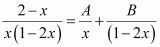Substituting x = 0 and in equation (1), we obtain

= 2 and B = 3

Substituting in equation (1), we obtain

Question 7:

Let

Equating the coefficients of x2x, and constant term, we obtain

A + C = 0

A + B = 1

B + C = 0

On solving these equations, we obtain

From equation (1), we obtain

Question 8:

Let

Substituting x = 1, we obtain

Equating the coefficients of x2 and constant term, we obtain

A + C = 0

−2A + 2B + C = 0

On solving, we obtain

Question 9:

Let

Substituting x = 1 in equation (1), we obtain

B = 4

Equating the coefficients of x2 and x, we obtain

A + C = 0

B − 2C = 3

On solving, we obtain

Question 10:

Let

Equating the coefficients of x2 and x, we obtain

Question 11:

LetSubstituting = −1, −2, and 2 respectively in equation (1), we obtain

Question 12:

It can be seen that the given integrand is not a proper fraction.

Therefore, on dividing (x3 + x + 1) by x2 − 1, we obtain

Let

Substituting = 1 and −1 in equation (1), we obtain

Question 13:

Equating the coefficient of x2x, and constant term, we obtain

A − B = 0

B − C = 0

A + C = 2

On solving these equations, we obtain

A = 1, B = 1, and C = 1

Question 14:

Equating the coefficient of x and constant term, we obtain

A = 3

2A + = −1 ⇒ B = −7

Question 15:

Equating the coefficient of x3x2, x, and constant term, we obtain

On solving these equations, we obtain

Question 16:

[Hint: multiply numerator and denominator by  and put xn = t]

Multiplying numerator and denominator by , we obtain

Substituting t = 0, −1 in equation (1), we obtain

A = 1 and B = −1

Question 17:

[Hint: Put sin x = t]

Substituting t = 2 and then t = 1 in equation (1), we obtain

A = 1 and B = −1

Question 18:

Equating the coefficients of x3x2x, and constant term, we obtain

A + C = 0

B + D = 4

4A + 3C = 0

4B + 3D = 10

On solving these equations, we obtain

A = 0, B = −2, C = 0, and D = 6

*Question 19:

Let x2 = t

⇒ 2x dx = dt

Substituting = −3 and = −1 in equation (1), we obtain

*Question 20:

Multiplying numerator and denominator by x3, we obtain

Let x4 = t

⇒ 4x3dx = dt

Substituting t = 0 and 1 in (1), we obtain

A = −1 and B = 1

*Question 21:

[Hint: Put ex = t]

Let ex =

⇒ ex dx = dt

Substituting t = 1 and t = 0 in equation (1), we obtain

A = −1 and B = 1

*Question 22:

A.  B.

C.  D.

Substituting x = 1 and 2 in (1), we obtain

A = −1 and B = 2

Hence, the correct answer is B.

*Question 23:

A.  B.

C.  D.

Equating the coefficients of x2x, and constant term, we obtain

A + B = 0

C = 0

A = 1

On solving these equations, we obtain

= 1, B = −1, and C = 0

Hence, the correct answer is A.

Exercise-7.6

Question 1:

x sin x

Let

Taking x as first function and sin x as second function and integrating by parts, we obtain

Question 2:

Let

Taking x as first function and sin 3x as second function and integrating by parts, we obtain

Question 3:

Let

Taking x2 as first function and ex as second function and integrating by parts, we obtain

Again, integrating by parts, we obtain

Question 4:

x logx

Let

Taking log x as first function and x as second function and integrating by parts, we obtain

Question 5:

x log 2x

Let

Taking log 2x as first function and x as second function and integrating by parts, we obtain

Question 6:

xlog x

Let

Taking log x as first function and x2 as second function and integrating by parts, we obtain

Question 7:

Let

Taking as first function and x as second function and integrating by parts, we obtain

Question 8:

Let

Taking as first function and x as second function and integrating by parts, we obtain

Question 9:

Let

Taking cos−1 x as first function and x as second function and integrating by parts, we obtain

Question 10:

Let

Taking as first function and 1 as second function and integrating by parts, we obtain

Question 11:

Let

Taking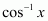as first function and as second function and integrating by parts, we obtain

Question 12:

Let

Taking x as first function and sec2x as second function and integrating by parts, we obtain

Question 13:

Let

Taking  as first function and 1 as second function and integrating by parts, we obtain

Question 14:

Taking  as first function and x as second function and integrating by parts, we obtain

Again, integrating by parts, we obtain

Question 15:

Let

Let I = I1 + I2 … (1)

Where,

and

Taking log x as first function and xas second function and integrating by parts, we obtain

Taking log x as first function and 1 as second function and integrating by parts, we obtain

Using equations (2) and (3) in (1), we obtain

Question 16:

Let

Let

⇒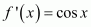∴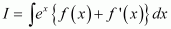It is known that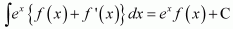Question 17:

Let

Let⇒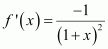It is known that,

Question 18:

Let

It is known that,

From equation (1), we obtain

Question 19:

Also, let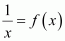It is known that,

*Question 20:

Let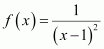⇒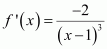It is known that,

*Question 21:

Let

Integrating by parts, we obtain

Again, integrating by parts, we obtain

*Question 22:

Let

= 2θ

Integrating by parts, we obtain

*Question 23:

equals

Let

Also, let

⇒Hence, the correct answer is A.

*Question 24:

equals

Let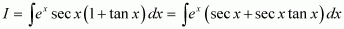Also, let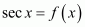⇒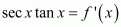It is known that,

Hence, the correct answer is B.

Exercise-7.7

Question 1:

Question 2:

Question 3:

Question 4:

Question 5:

Question 6:

Question 7:

*Question 8:

*Question 9:

*Question 10:

is equal to

A.  B.

C.  D.

Hence, the correct answer is A.

*Question 11:

is equal to

A.

B.

C.

D.

Hence, the correct answer is D.

Exercise-7.8

Question 1:

It is known that,

Question 2:

It is known that,

Question 3:

It is known that,

Question 4:

It is known that,

From equations (2) and (3), we obtain

Question 5:

It is known that,

Question 6:

It is known that,

Exercise-7.9

Question 1:

By second fundamental theorem of calculus, we obtain

Question 2:

By second fundamental theorem of calculus, we obtain

Question 3:

By second fundamental theorem of calculus, we obtain

Question 4:

By second fundamental theorem of calculus, we obtain

Question 5:

By second fundamental theorem of calculus, we obtain

Question 6:

By second fundamental theorem of calculus, we obtain

Question 7:

By second fundamental theorem of calculus, we obtain

Question 8:

By second fundamental theorem of calculus, we obtain

Question 9:

By second fundamental theorem of calculus, we obtain

Question 10:

By second fundamental theorem of calculus, we obtain

Question 11:

By second fundamental theorem of calculus, we obtain

Question 12:

By second fundamental theorem of calculus, we obtain

Question 13:

By second fundamental theorem of calculus, we obtain

Question 14:

By second fundamental theorem of calculus, we obtain

Question 15:

By second fundamental theorem of calculus, we obtain

Question 16:

Let

Equating the coefficients of x and constant term, we obtain

A = 10 and B = −25

Substituting the value of I1 in (1), we obtain

Question 17:

By second fundamental theorem of calculus, we obtain

Question 18:

By second fundamental theorem of calculus, we obtain

*Question 19:

By second fundamental theorem of calculus, we obtain

*Question 20:

By second fundamental theorem of calculus, we obtain

*Question 21:

equals

A.  B.  C.  D.

By second fundamental theorem of calculus, we obtain

Hence, the correct answer is D.

*Question 22: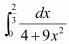equals

A.  B.  C.  D.

By second fundamental theorem of calculus, we obtain

Hence, the correct answer is C.

Exercise-7.10

Question 1:

When x = 0, t = 1 and when x = 1, t = 2

Question 2:

Also, let

Question 3:

Also, let x = tanθ

⇒ dx = sec2θ dθ

When x = 0, θ = 0 and when = 1,

Takingas first function and sec2θ as second function and integrating by parts, we obtain

Question 4:

Let + 2 = t2

⇒ dx = 2tdt

When x = 0,  and when = 2, = 2

Question 5:

Let cos x = t

⇒ −sinx dx = dt

When x = 0, = 1 and when

Question 6:

Let

⇒ dx = dt

*Question 7:

Let x + 1 =

⇒ dx = dt

When x = −1, = 0 and when x = 1, = 2

*Question 8:

Let 2x = t

⇒ 2dx = dt

When x = 1, t = 2 and when x = 2, t = 4

*Question 9:

The value of the integral is

A. 6 B. 0 C. 3 D. 4

Let cotθ = t

⇒ −cosec2θ dθdt

Hence, the correct answer is A.

*Question 10:

If  is

A.  cos x + x sin x

B.  x sin x

C.  x cos x

D.  sin x cos x

Integrating by parts, we obtain

Hence, the correct answer is B.

Exercise-7.11

Question 1:

Adding (1) and (2), we obtain

Question 2:

Adding (1) and (2), we obtain

Question 3:

Adding (1) and (2), we obtain

Question 4:

Adding (1) and (2), we obtain

Question 5:

It can be seen that (x + 2) ≤ 0 on [−5, −2] and (x + 2) ≥ 0 on [−2, 5].

Question 6:

It can be seen that (x − 5) ≤ 0on [2, 5] and (x − 5) ≥ 0 on [5, 8].

Question 7:

Question 8:

Question 9:

Question 10:

Adding (1) and (2), we obtain

Question 11:

As sin(−x) = (sin (−x))2 = (−sin x)2 = sin2x, therefore, sin2is an even function.

It is known that if f(x) is an even function, then

Question 12:

Adding (1) and (2), we obtain

Question 13:

As sin(−x) = (sin (−x))7 = (−sin x)7 = −sin7x, therefore, sin2is an odd function.

It is known that, if f(x) is an odd function, then

Question 14:

It is known that,

Question 15:

Adding (1) and (2), we obtain

Question 16:

Adding (1) and (2), we obtain

sin (π − x) = sin x

Adding (4) and (5), we obtain

Using by parts

*Question 17:

It is known that,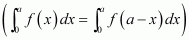Adding (1) and (2), we obtain

*Question 18:

It can be seen that, (x − 1) ≤ 0 when 0 ≤ x ≤ 1 and (x − 1) ≥ 0 when 1 ≤ x ≤ 4

*Question 19:

Show that if f and g are defined as and

Adding (1) and (2), we obtain

*Question 20:

The value of is

A. 0 B. 2 C. Π D. 1

It is known that if f(x) is an even function, then and

if f(x) is an odd function, then

Hence, the correct answer is C.

*Question 21:

The value of is

A. 2 B.  C. 0 D.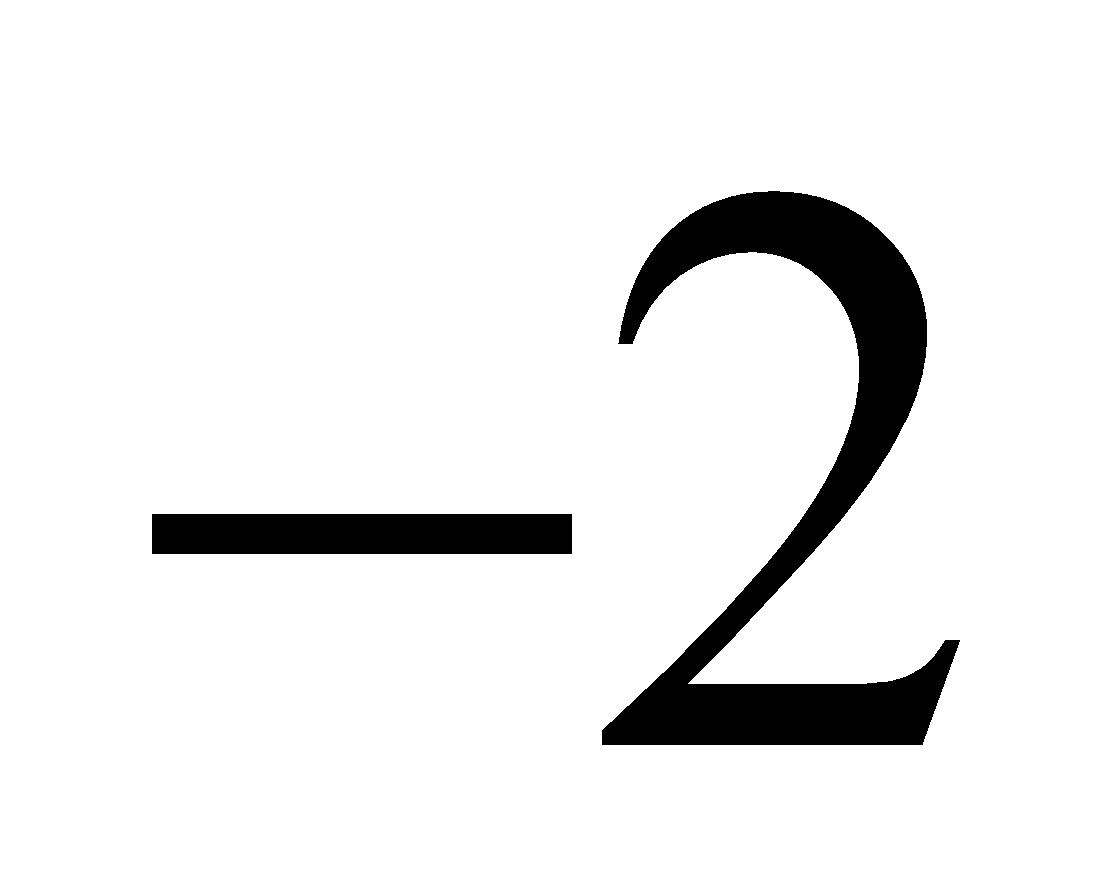Adding (1) and (2), we obtain

Hence, the correct answer is C.

Miscellaneous Exercise

*Question1:

Equating the coefficients of x2x, and constant term, we obtain

A + B − C = 0

B + = 0

A = 1

On solving these equations, we obtain

From equation (1), we obtain

*Question 2:

*Question 3:

[Hint: Put]

*Question 4:

*Question 5:

On dividing, we obtain

*Question 6:

Equating the coefficients of x2x, and constant term, we obtain

A + B = 0

C = 5

9A + = 0

On solving these equations, we obtain

From equation (1), we obtain

*Question 7:

Let  a t

⇒ dx = dt

*Question 8:

*Question 9:

Let sin x = t

⇒ cos x dx = dt

*Question 10:

*Question 11:

*Question 12:

Let x= t

⇒ 4x3 dx = dt

*Question 13:

Let ex = t

⇒ ex dx = dt

*Question 14:

Equating the coefficients of x3x2x, and constant term, we obtain

A + C = 0

B + D = 0

4A + C = 0

4D = 1

On solving these equations, we obtain

From equation (1), we obtain

*Question 15: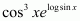= cos3 x × sin x

Let cos x = t

⇒ −sin x dx = dt

*Question 16:

*Question 17:

*Question 18:

*Question 19:

….(i)

= taking

Let

∴ Integral

….(2)

From (1) and (2)

*Question 20:

*Question 21:

*Question 22:

Equating the coefficients of x2x,and constant term, we obtain

A + C = 1

3A + B + 2= 1

2A + 2B + C = 1

On solving these equations, we obtain

A = −2, B = 1, and C = 3

From equation (1), we obtain

*Question 23:

*Question 24:

Integrating by parts, we obtain

*Question 25:

*Question 26:

When = 0, = 0 and  when

*Question 27:

When and when

*Question 28:

When and when

As  therefore, is an even function.

It is known that if f(x) is an even function, then

*Question 29:

*Question 30:

*Question 31:

From equation (1), we obtain

*Question 32:

Adding (1) and (2), we obtain

*Question 33:

From equations (1), (2), (3), and (4), we obtain

*Question 34:

Equating the coefficients of x2x, and constant term, we obtain

A + C = 0

A + B = 0

B = 1

On solving these equations, we obtain

A = −1, C = 1, and B = 1

Hence, the given result is proved.

*Question 35:

Integrating by parts, we obtain

Hence, the given result is proved.

*Question 36:

Therefore, f (x) is an odd function.

It is known that if f(x) is an odd function, then

Hence, the given result is proved.

*Question 37:

Hence, the given result is proved.

*Question 38:

Hence, the given result is proved.

*Question 39:

Integrating by parts, we obtain

Let 1 − x2 = t

⇒ −2x dx = dt

Hence, the given result is proved.

*Question 40:

Evaluate as a limit of a sum.

It is known that,

*Question 41:

is equal to

A.  B.

C.  D.

Hence, the correct answer is A.

*Question 42:

is equal to

A.  B.

C.  D.

Hence, the correct answer is B.

*Question 43:

If then is equal to

A.  B.

C.  D.

Hence, the correct answer is D.

*Question 44:

The value of is

A. 1 B. 0 C.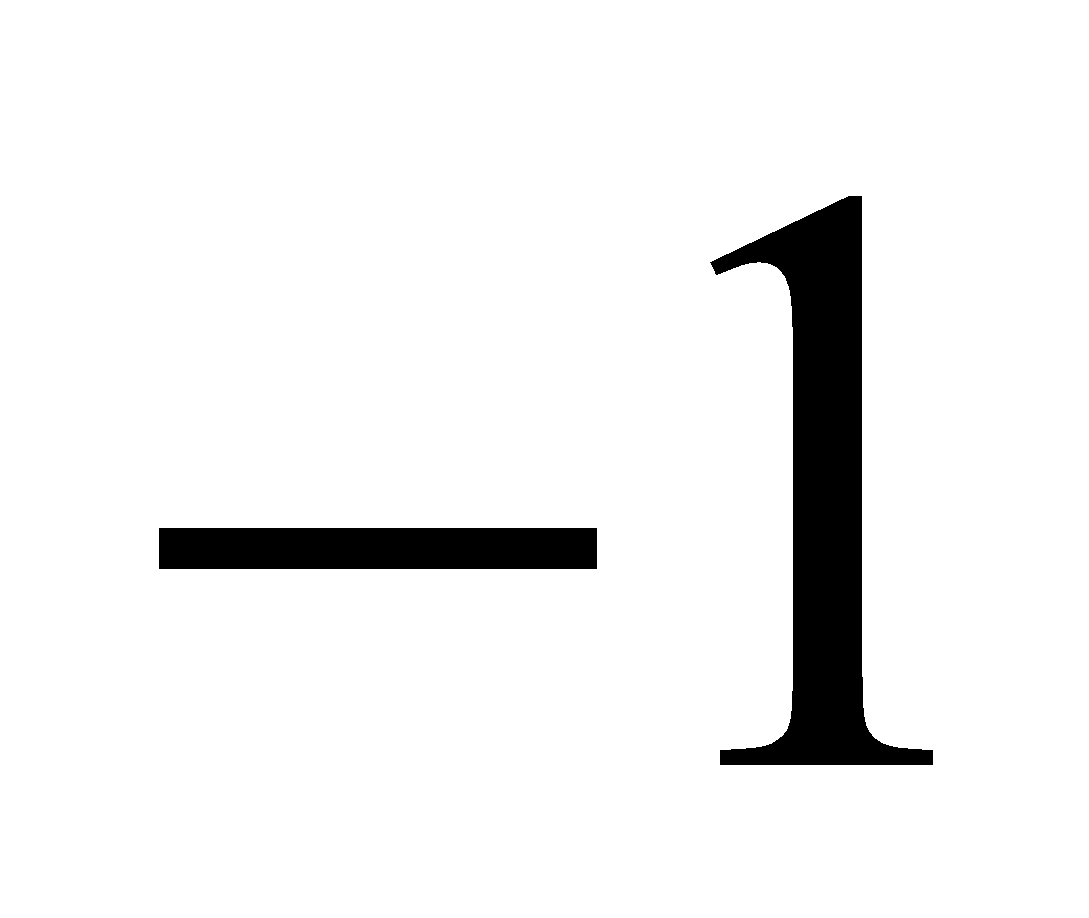D.

Adding (1) and (2), we obtain

Hence, the correct answer is B.

Conclusion Swastik Classes’ NCERT Solution for Class 12 Mathematics Chapter 7, “Integrals,” provides a comprehensive study material that helps students understand the fundamental concepts of integral calculus. The solutions offer step-by-step explanations and solved examples that help students develop a deeper understanding of the subject. The chapter covers various topics, including properties of integrals, methods of integration, integration using partial fractions, and integration using trigonometric identities. With the help of these solutions, students can improve their problem-solving skills and gain the confidence to tackle complex integral problems. Swastik Classes’ NCERT solutions are designed according to the latest CBSE syllabus, making them useful for students preparing for board exams or competitive exams like JEE and NEET. Overall, Swastik Classes’ NCERT Solution for Class 12 Mathematics Chapter 7 is an excellent resource for students who want to excel in mathematics and build a strong foundation in integral calculus.

### What is the meaning of integrals in NCERT Solutions for Class 12 Maths Chapter 7?

The area under a curve is often referred to as integral in Calculus. It can be represented on a graph, as a mathematical function. List of important formulas for determining the integration of various functions is covered in this chapter. Integral is also known as antiderivative and we also know that integration formulas of some functions are the reverse of their differentiation formulas. This chapter is also considered to be one of the important chapters from the exam point of view.

### Is Chapter 7 of NCERT Solutions for Class 12 Maths difficult to understand?

No, Chapter 7 of NCERT Solutions for Class 12 Maths is not difficult to understand. It is one of the interesting topics in Class 12 which would be continued in higher levels of education also. Good knowledge of integral formulas will make it easier for the students to solve the basic sums of integration efficiently. A proper knowledge of derivatives is necessary to understand the concepts of integral calculus without any difficulty.

### Where can I download the NCERT Solutions for Class 12 Maths Chapter 7 PDF online?

You can find the NCERT Solutions for Class 12 Maths Chapter 7 on SWC. The solutions are prepared by the highly experienced faculty having vast conceptual knowledge. These are considered to be one of the best rated solutions available online. All the problems from the NCERT textbook are solved in a step wise manner based on the latest syllabus and exam pattern designed by the CBSE board.